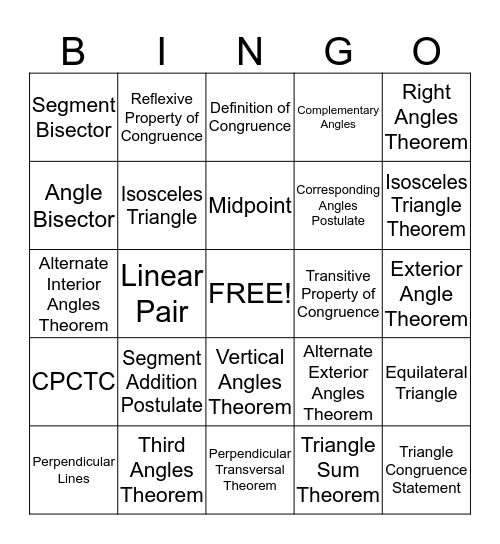# Proof Proptery BingoThis bingo card has a free space and 24 words: Segment Addition Postulate, Third Angles Theorem, Midpoint, Perpendicular Lines, Right Angles Theorem, Segment Bisector, Angle Bisector, Reflexive Property of Congruence, Transitive Property of Congruence, Vertical Angles Theorem, Corresponding Angles Postulate, Alternate Interior Angles Theorem, Alternate Exterior Angles Theorem, Equilateral Triangle, Isosceles Triangle, Triangle Sum Theorem, Exterior Angle Theorem, Definition of Congruence, Isosceles Triangle Theorem, Linear Pair, Triangle Congruence Statement, CPCTC, Complementary Angles and Perpendicular Transversal Theorem.

## Play Online

Share this URL with your players:For more control of your online game, create a clone of this card first.

## Probabilities

With players vying for a you'll have to call about __ items before someone wins. There's a __% chance that a lucky player would win after calling __ items.

Tip: If you want your game to last longer (on average), add more unique words/images to it.